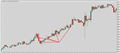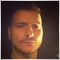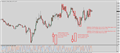Candle body alert indicator72

Hello community,

I was wondering if someone could help me write simple full candle body alert indicator. This would be alert indicator (with poped up alert display+sound- standard of Mt4). Indicator would find candle with wicks on up and down that are less than X % of full candle size. Prefered would be 10% or less.

3 TFs per chart to watch.

For example this means that if candle like that would appear, indicator would give alert signal:

http://prntscr.com/4xnhq9

I would appreatate help.Screenshot
• prnt.sc
Captured with Lightshot32776

janpec1000:

Hello community,

I was wondering if someone could help me write simple full candle body alert indicator. This would be alert indicator (with poped up alert display+sound- standard of Mt4). Indicator would find candle with wicks on up and down that are less than X % of full candle size. Prefered would be 10% or less.

3 TFs per chart to watch.

For example this means that if candle like that would appear, indicator would give alert signal:

http://prntscr.com/4xnhq9

I would appreatate help.

Both wicks combined , each wick separately?
If you run the calculation separately , and both are 10% or less which signal are you going with?72

LoRio:
Both wicks combined , each wick separately?
If you run the calculation separately , and both are 10% or less which signal are you going with?

If you could make X allowed lenght for each side that would be great.

It doesnt matter which signal it is, it is only 1 signal.72

Could you perhaps deliver this by end of today, i would really appretiate it since it would be needed in tomorrows trading.32776

janpec1000:
Could you perhaps deliver this by end of today, i would really appretiate it since it would be needed in tomorrows trading.
Sure . i have a break now , i'll give it a shot.32776

//+------------------------------------------------------------------+
//|                                                        Teddy.mq4 |
//|                        Copyright 2014, MetaQuotes Software Corp. |
//|                                              http://www.mql5.com |
//+------------------------------------------------------------------+
#property copyright "Copyright 2014, MetaQuotes Software Corp."
#property version   "1.00"
#property strict
#property indicator_separate_window
#property indicator_buffers 4
#property indicator_plots   4
//--- plot
#property indicator_label1  "upwick %"
#property indicator_type1  DRAW_LINE
#property indicator_color1  clrDodgerBlue
#property indicator_style1  STYLE_SOLID
#property indicator_width1  1

//--- plot
#property indicator_label2  "downwick%"
#property indicator_type2   DRAW_LINE
#property indicator_color2  clrOrangeRed
#property indicator_style2  STYLE_SOLID
#property indicator_width2  1
//--- plot
#property indicator_label3  "up conf"
#property indicator_type3  DRAW_NONE
#property indicator_width3  1

//--- plot
#property indicator_label4  "down conf"
#property indicator_type4   DRAW_NONE
#property indicator_width4  1

input int Up_Wick=10;//Up Wick %
input int Dw_Wick=10;//Dw Wick %
enum Teds_Method
{
};

string Teds_Code="x";
double uwBuffer[];
double dwBuffer[];
double upConf[];
double dwConf[];
double T_Pip_Size=0;
//+------------------------------------------------------------------+
//| Custom indicator initialization function                         |
//+------------------------------------------------------------------+
int OnInit()
{
if(Digits()==2||Digits()==3)
{
T_Pip_Size=0.01;
}
if(Digits()==4||Digits()==5)
{
T_Pip_Size=0.0001;
}
SetIndexBuffer(0,uwBuffer);
SetIndexBuffer(1,dwBuffer);
SetIndexBuffer(2,upConf);
SetIndexBuffer(3,dwConf);
//--- indicator buffers mapping
Teds_Code="x";
//---
return(INIT_SUCCEEDED);
}
//+------------------------------------------------------------------+
//| Custom indicator iteration function                              |
//+------------------------------------------------------------------+
int OnCalculate(const int rates_total,
const int prev_calculated,
const datetime &time[],
const double &open[],
const double &high[],
const double &low[],
const double &close[],
const long &tick_volume[],
const long &volume[],
{
//---
int i,pos,limit;
pos=prev_calculated-1;
limit=rates_total;
if(limit>2)
{
limit=limit-5;
}
if(pos<0)
{
pos=0;
}
double cs,uw,dw,unit;
for(i=pos; i<=limit && !IsStopped(); i++)
{
//get candle size
cs=Open[i]-Close[i];
cs=MathAbs(cs/T_Pip_Size);
if(Open[i]>=Close[i])
{
uw=High[i]-Open[i];
dw=Close[i]-Low[i];
}
if(Open[i]<Close[i])
{
uw=High[i]-Close[i];
dw=Open[i]-Low[i];
}
//get unit
unit=cs/100;
if(unit!=0)
{
//upwick percentage
uw=(uw/T_Pip_Size)/unit;
//downwick percentage
dw=(dw/T_Pip_Size)/unit;
if(uw>100)
{
uw=100;
}
if(dw>100)
{
dw=100;
}
uwBuffer[i]=uw;
dwBuffer[i]=dw;
upConf[i]=1;
dwConf[i]=1;
}
if(unit==0)
{
uwBuffer[i]=0;
dwBuffer[i]=0;
}
}
string now_code=Open+"::"+High+"::"+Low+"::"+Close+"::"+Volume;
if(now_code!=Teds_Code)
{
{
if(uwBuffer<=Up_Wick&&dwBuffer<=Dw_Wick&&upConf==1&&dwConf==1)
{
}
}
{
if((uwBuffer<=Up_Wick||dwBuffer<=Dw_Wick)&&upConf==1&&dwConf==1)
{
}
}
Teds_Code=now_code;
}
//--- return value of prev_calculated for next call
return(rates_total);
}
//+------------------------------------------------------------------+
Here72

LoRio:
Here

Thanks a lot i will reply how it goes tomorrow.32776

janpec1000:

Thanks a lot i will reply how it goes tomorrow.

:) enjoy72

Hey could you fix alert to work properly. At moment it doesnt give alerts if you have it opened on chart, only if i switch in between TFs it will create alert. The problem is once 2 alerts sign and alert window dissapears, it no longer triggeres alerts.32776

//+------------------------------------------------------------------+
//|                                                        Teddy.mq4 |
//|                        Copyright 2014, MetaQuotes Software Corp. |
//|                                              http://www.mql5.com |
//+------------------------------------------------------------------+
#property copyright "Copyright 2014, MetaQuotes Software Corp."
#property version   "1.00"
#property strict
#property indicator_separate_window
#property indicator_buffers 4
#property indicator_plots   4
//--- plot
#property indicator_label1  "upwick %"
#property indicator_type1  DRAW_LINE
#property indicator_color1  clrDodgerBlue
#property indicator_style1  STYLE_SOLID
#property indicator_width1  1

//--- plot
#property indicator_label2  "downwick%"
#property indicator_type2   DRAW_LINE
#property indicator_color2  clrOrangeRed
#property indicator_style2  STYLE_SOLID
#property indicator_width2  1
//--- plot
#property indicator_label3  "up conf"
#property indicator_type3  DRAW_NONE
#property indicator_width3  1

//--- plot
#property indicator_label4  "down conf"
#property indicator_type4   DRAW_NONE
#property indicator_width4  1

input int Up_Wick=10;//Up Wick %
input int Dw_Wick=10;//Dw Wick %
enum Teds_Method
{
};

string Teds_Code="x";
double uwBuffer[];
double dwBuffer[];
double upConf[];
double dwConf[];
double T_Pip_Size=0;
//+------------------------------------------------------------------+
//| Custom indicator initialization function                         |
//+------------------------------------------------------------------+
int OnInit()
{
if(Digits()==2||Digits()==3)
{
T_Pip_Size=0.01;
}
if(Digits()==4||Digits()==5)
{
T_Pip_Size=0.0001;
}
SetIndexBuffer(0,uwBuffer);
SetIndexBuffer(1,dwBuffer);
SetIndexBuffer(2,upConf);
SetIndexBuffer(3,dwConf);
//--- indicator buffers mapping
Teds_Code="x";
//---
return(INIT_SUCCEEDED);
}
//+------------------------------------------------------------------+
//| Custom indicator iteration function                              |
//+------------------------------------------------------------------+
int OnCalculate(const int rates_total,
const int prev_calculated,
const datetime &time[],
const double &open[],
const double &high[],
const double &low[],
const double &close[],
const long &tick_volume[],
const long &volume[],
{
//---
int i,pos,limit;
pos=prev_calculated-1;
limit=rates_total;
if(limit>2)
{
limit=limit-5;
}
if(pos<0)
{
pos=0;
}
double cs,uw,dw,unit;
for(i=pos; i<=limit && !IsStopped(); i++)
{
//get candle size
cs=Open[i]-Close[i];
cs=MathAbs(cs/T_Pip_Size);
if(Open[i]>=Close[i])
{
uw=High[i]-Open[i];
dw=Close[i]-Low[i];
}
if(Open[i]<Close[i])
{
uw=High[i]-Close[i];
dw=Open[i]-Low[i];
}
//get unit
unit=cs/100;
if(unit!=0)
{
//upwick percentage
uw=(uw/T_Pip_Size)/unit;
//downwick percentage
dw=(dw/T_Pip_Size)/unit;
if(uw>100)
{
uw=100;
}
if(dw>100)
{
dw=100;
}
uwBuffer[i]=uw;
dwBuffer[i]=dw;
upConf[i]=1;
dwConf[i]=1;
}
if(unit==0)
{
uwBuffer[i]=0;
dwBuffer[i]=0;
}
}
//get candle size
cs=Open-Close;
cs=MathAbs(cs/T_Pip_Size);
if(Open>=Close)
{
uw=High-Open;
dw=Close-Low;
}
if(Open<Close)
{
uw=High-Close;
dw=Open-Low;
}
//get unit
unit=cs/100;
if(unit!=0)
{
//upwick percentage
uw=(uw/T_Pip_Size)/unit;
//downwick percentage
dw=(dw/T_Pip_Size)/unit;
if(uw>100)
{
uw=100;
}
if(dw>100)
{
dw=100;
}
uwBuffer=uw;
dwBuffer=dw;
upConf=1;
dwConf=1;
}
if(unit==0)
{
uwBuffer=0;
dwBuffer=0;
}
string now_code=Open+"::"+High+"::"+Low+"::"+Close+"::"+Volume;
if(now_code!=Teds_Code)
{
{
if(uwBuffer<=Up_Wick&&dwBuffer<=Dw_Wick&&upConf==1&&dwConf==1)
{
}
}
{
if((uwBuffer<=Up_Wick||dwBuffer<=Dw_Wick)&&upConf==1&&dwConf==1)
{
}
}
Teds_Code=now_code;
}
//--- return value of prev_calculated for next call
return(rates_total);
}
//+------------------------------------------------------------------+
sure72

Thank you very much for your help. Indicator is working ok now.

I know i am probably pushing too far as you are totaly helping for free, but i was thinking maybe if you could also help me on pinbar break alert indicator. This is last request i would have.

http://prntscr.com/4y06q6

Principle is that when price goes into supply (high) and there is bullish pinbar spawned, and next candle breaks it its bearish signal, as lows on lower Tf get broken signaling strenght of supply and short signal. Its oposite for long signal in term of demand.

If you could make this indicator same being alert one as previous one it would be great, becouse its really hard to track candle per candle otherwise such strategy and having alerts is much better.Screenshot
• prnt.sc
Captured with Lightshot